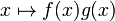Pointwise combination of functions

For instance, pointwise sum of functions$f$ and$g$ is the function$x \mapsto f(x) + g(x)$. Similarly, pointwise product of functions$f$ and$g$ is thee function$x \mapsto f(x)g(x)$.